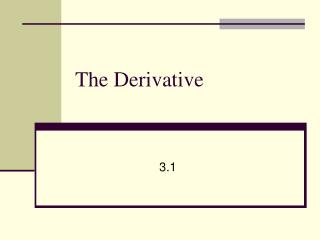DownloadDownload PresentationThe Derivative

# The Derivative

Télécharger la présentation## The Derivative

- - - - - - - - - - - - - - - - - - - - - - - - - - - E N D - - - - - - - - - - - - - - - - - - - - - - - - - - -
##### Presentation Transcript

1. Calculus Differential Calc Integral Calc

2. Derivative – instantaneous rate of change of one variable wrt another. Differentiation – process of finding the derivative

3. Finding Average Rate of Change • A piece of chocolate is pulled from a refrigerator (6° C) and placed on a counter (22° C). The temperature of the chocolate is given by: What is the average rate of change in the temperature of the chocolate from 8 to 20 minutes? The rate of change was not constant thru out the process. This only tell us what happened on average over a period of time!

4. y (b, f(b)) (a, f(a)) a b x Instantaneous Rate of Change We take the limit of the average rate of change as we let the intervals get smaller and smaller ∆x  0 Tangent Line

5. Tangent Line Definition of Tangent Line The tangent line to the graph of y = f(x) at x = c is the line through the point (c, f(c)) with slope provided this limit exists. If the instantaneous rate of change of f(x) with respect to x exists at a point c, then it is the slope of the tangent line at that point.

6. Find the slope of the tangent line at x = 3 if

7. The derivative is same as the slope of the tangent line Derivative at any point To Find slope of tangent line at a given point. Plug given point in f’(x)

8. “the derivative of f with respect to x” “f prime x” or “y prime” “the derivative of y with respect to x” or “dee why dee ecks” “the derivative of f with respect to x” or “dee eff dee ecks” “the derivative of f of x” “dee dee ecks uv eff uv ecks” or

9. The derivative is the slope of the original function. The derivative is defined at the end points of a function on a closed interval.

10. Find the derivative of f(x) and use it to find the equation of the tangent line at the point x = 4 Slope: -19 and point (4, -28)

11. Find dy/dx for